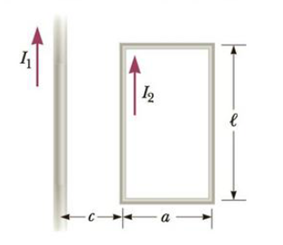Chapter 19, Problem 58P

Chapter
Section
Textbook Problem

In Figure P19.58 the current in the long, straight wire is I1 = 5.00 A, and the wire lies in the plane of the rectangular loop, which carries 10.0 A. The dimensions shown are c = 0.100 m, a = 0.150 m, and ℓ = 0.450 m. Find the magnitude and direction of the net force exerted by the magnetic field due to the straight wire on the loop.Figure P19.58

To determine
The magnitude and direction of the net force exerted by the magnetic field due to the straight wire on the rectangular loop.

Explanation

Given info: The current in long straight wire is I1=5.00A . The current in the rectangular loop wire is 10.0A . c=0.100m , a=0.150m and l=0.450m .

Explanation:

In the rectangular loop, the magnetic force on the top and bottom segments will cancel each other as they are equal in magnitude and opposite in direction. Hence only the left and right side of the loop are to be taken into consideration.

The force between two parallel long straight current carrying wire is given by,

F=μ0I1I2l2πd

• F is the force
• I1 is the current in the top wire
• I2 is the current in the bottom wire
• d is the distance between the wires
• μ0 is the permeability of free space

Choose the left side as the positive direction.

The net force on the left and the right arm of the rectangular loop will be,

Fnet=μ0I1I2l2πcμ0I1I2l2π(c+a)=μ0I1I2l2π(1c1c+a)

Substitute 4π×107TmA-1 for μ0 , 5

Still sussing out bartleby?

Check out a sample textbook solution.

See a sample solution

The Solution to Your Study Problems

Bartleby provides explanations to thousands of textbook problems written by our experts, many with advanced degrees!

Get Started